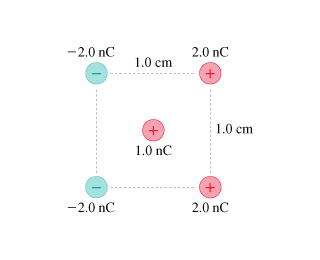# Problem: What is the force F on the 1.0 nC charge in the middle of the figure due to the four other charges? Give your answer in component form.Assume that x-axis is directed horizontally to the right, and y-axis is directed vertically upward.

###### FREE Expert Solution

The charges on the upper part and the lower-left produce a force, which points towards the lower left.

The remaining two charges produce a force in the upper left direction.

Because of symmetry, these two forces have equal magnitude.

The distance to the center is given by:

dc = sqrt(2)(0.005) = 7.07 × 10-3.

80% (33 ratings)###### Problem Details

What is the force F on the 1.0 nC charge in the middle of the figure due to the four other charges? Give your answer in component form.

Assume that x-axis is directed horizontally to the right, and y-axis is directed vertically upward.